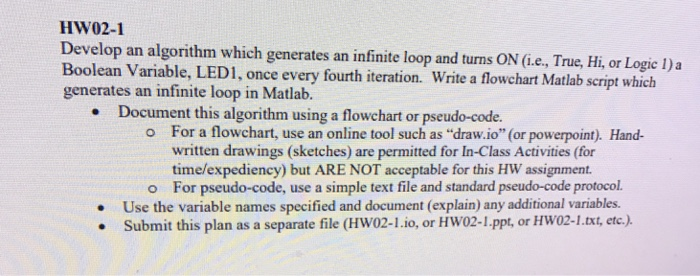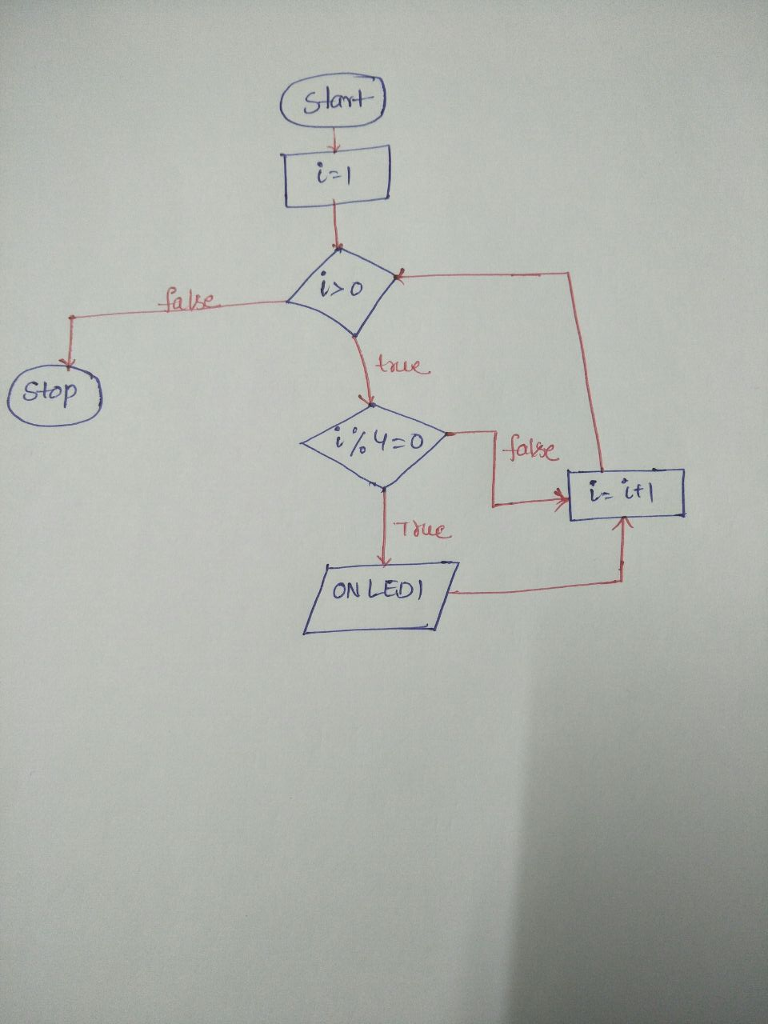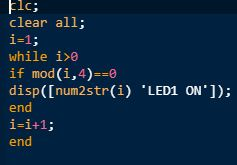# Homework Solution: Develop an algorithm which generates an infinite loop…In matlab
Develop an algorithm which generates an infinite loop and turns ON (i.e., True, Hi, or Logic 1)a Boolean Variable, LED 1, once every fourth iteration. Write a flowchart Matlab script which generates an infinite loop in Matlab. Document this algorithm using a flowchart or pseudo-code. For a flowchart, use an online tool such as "draw, io" (or powerpoint). Handwritten drawings (sketches) are permitted for In-Class Activities (for time/expediency) but ARE NOT acceptable for this HW assignment. For pseudo-code, use a simple text file and standard pseudo-code protocol. Use the variable names specified and document (explain) any additional variables. Submit this plan as a separate file (HW02-1.io, or HW02-1.ppt, or HW02-1.txt, etc.).